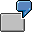Example: Quantity DeterminationStarting Situation

You use a flexible function to determine a specific plan quantity can be determined through the template. Example: the material transport from inventory to production site in a new plant is a source of overhead costs, which until now have been calculated only on a percentage basis. The template is to assign an exact measure of overhead cost in this scenario. The process driver for this is the number of pallet movements for a certain production lot size, so the template is to calculate the number based on the production lot size. In this example, remember the larger production components require more pallet movements than smaller ones. Also keep in mind that a pallet movement is necessary even if only one component is required.

The following data is assumed to be created. The information in parentheses is for the data required by IDES.

• A material master record, to which a BOM is assigned, the master record and each component on the BOM must be maintained for the same alternative unit of measure (PAL)
• Function TotalOfBOMItems, total of formula results at the level of BOM items for all of them.
• Function Ceil, rounding up to the next whole figure
• Function BOMItemRequiredQuantity, required quantity of the material from the BOM items.
• Function AlternativeUnitOfMeasure, the packing unit of the component; that is, the unit of measure for which you determine a conversion factor
• Function MaterialNumber, material number
• Function BOMItemComponents, material number of the BOM item.

Procedure

Prerequisites

You have created an algorithm that calculates the number of pallet movements needed. It does the following:

• Calculate lot size x number of pieces according to the BOM item / factor of the alternative unit of measure (piece per pallet)
• Rounds up to the next whole figure

The end result is the number of pallet movements necessary for a component of the BOM.

• Finally, it totals the pallet movements of all components of the BOM.

This algorithm is now entered as the quantity formula of the template.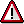If the alternative unit of measure has not been maintained, then do this next. This unit of measure must be the same for all components of the BOMs. In the following example, we create it for one component only: namely, material r-4001.

To view the alternative unit of measure in IDES only, then see "Alternative Unit of Measure in IDES".

Entry of Alternative Unit of measures

1. Choose Logistics ® Materials management ® Material master ® Material ® Change ® Immediately.
2. Enter the material number (r-4001).
3. Select Basic Data 1.
4. Choose the additional data Units of measure.
5. In the column titled AUn, enter the name of the alternative unit of measure (PAL).
6. In column Y enter the number of basis unit of measures to which the alternative unit of measure corresponds.
7. Save your entries.

Results of this Step

The alternative unit of measure has been created. The IDES example indicates the unit PAL (pallets). Column Y indicates the conversion factor "basis units of measure - alternative unit measure", which is the number of pieces per pallet in the example.

In the next step, enter the quantity formula from the algorithm into the column Quantity Plan in the template.

Enter Quantity Formula

If you wish to only view the alternative unit of measure in IDES, see "Alternative Unit of Measure in IDES".

1. Call up the template for editing.
2. Double click on column Plan qty.
3. The function selection opens at the lower right of the screen. Choose the function TotalOfBOMItems (BOM node) by dragging it to the left into the editor using Drag&Drop.
4. This function totals the necessary pallet movements of all BOM items.

5. The narrow screen area Flexible Function Condition is displayed. Choose Flexible Function Formula.
6. Choose the Ceil function (node Mathematical Functions) from the function selection.
7. This function rounds up the result to the next whole number.

8. In the editor, choose the button for an opening parenthesis.
9. Choose the function BOMItemRequiredQuantity (node: BOM).
10. This function provides you with the required quantity per BOM item; in the IDES example, you are provided with the number of pieces per BOM item (lot size x number of pieces per BOM item).

11. In the editor, choose the button for division (/).
12. Choose the function ALTQuantityFactorFromMaterial (node: Material).
13. This function provides you with the factor, which the result "lot size x number of pieces of the BOM components" will be divided by.

14. The system displays the Parameters for Function dialog box.
15. Row Unit of Measure, column Parameter Value: Use F4 to choose PAL (Pallet). This entry is necessary to arrive at the correct conversion factor (base unit of measure - alternative unit of measure).
16. Row Material Number, column Parameter Value: Double click on the row. The system displays the available functions.
17. Double-click on BOMItemComponents. The function is displayed in the Material Number row. The system finds the material number of the BOM item just processed.
18. Choose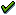Continue in the Parameters for Function dialog box. The flexible function formula is displayed in the editor.
19. In the editor, choose the button for a closing parenthesis.
20. Choose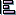Pretty Printer.
21. ChooseCheck Formula in the Editor.
22. In the Flexible Function Condition dialog box, chooseContinue.
23. In the editor, choosePlan Quantity.
24. Save the template.

Result

The quantity formula is now as follows:

CEIL (BOM_ITEM_REQUIRED_QUANTITY / ALT_QTY_FACTOR_FROM_MATERIAL (MATERIAL_NUMBER = BOM_ITEM_COMPONENTS , ALTERNATIVE_QUANTITY_UNIT = `PAL´ ).

Viewing The Alternative Unit of Measure in IDES

1. Choose Logistics ® Materials management ® Material master ® Material ® Display ® Display current status.
2. Enter the material number (r-4001).
3. Select Basic Data 1.
4. Choose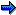Additional Data.
5. Choose the Units of Measure tab page.

In column AME you can see the alternative unit of measure (PAL), and in column Y the conversion factor.

Viewing The Quantity Formula in IDES

1. Call up the template for editing (T-PROD3, environment 001).
2. Double click on column Plan qty.
3. Position the cursor on \$1 in the editor.
4. In the Flexible Function Condition dialog box, choose Flexible Function Formula.

Here you see the entire quantity formula. Leave the function without saving.

Checking and Testing Templates

You are either on the Create template or Change template screen. To check the syntax of your entries, choose Template -> Check or.

Follow these steps to test the template:

1. Choose Template ® Test Plan or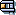.
2. Enter the test parameter in the IDES system; for example, ORDER_PLANT: 1200 and ORDER_MATERIAL_NUMBER: r-4050 .

IDES produces these results with the following entries in TOTAL_ORDER_QUANTITY:

 Entries in TOTAL_ORDER_QUANTITY Results (pallet movements) 1 7 10 8 50 14 100 22 1000 187

The results can be clarified as follows:

Seven components belong to material r-4050. The SAP system uses this calculation for the pallet movements.

Lot size (= Entry in TOTAL_ORDER_QUANTITY) * piece per material r-4050 / factor of the alternative unit of measure (piece per pallet). The result is rounded up to the next whole number. With a 100 piece order of material R-4050, the following results are obtained:

 Material number Quantity per material r-4050 Factor of the alternative unit of measure (= pieces per pallet) Pallet movements r-4001. 1 200 1 r-4002. 4 12.000 1 r-4003. 1 60 2 r-4004. 1 8 13 r-4005. 1 160 1 r-4006. 1 160 1 r-4007. 1 40 3 Total pallet movements 22

Template Evaluation

To see more details, double click on the Quantity row. You receive the following information:

Formula/Method: place holder for the name of the function.

Function List: the system informs you that this function works with a flexible function (\$1). The parameters of the function are listed (all are "invisible"). The last row shows the results of the calculation: 22 pallet movements.

Result: display total results (22).

Field content: the system shows, for example, the material number you entered.

Template Evaluation - Details

To see the calculation results, use the button Quantity Flexible Function and choose the defaulted function. The system returns the same information as in the above table: Field content shows the "Material number", Function list shows the "Factor of the alternative unit of measure", and Results shows the "Palette movements".